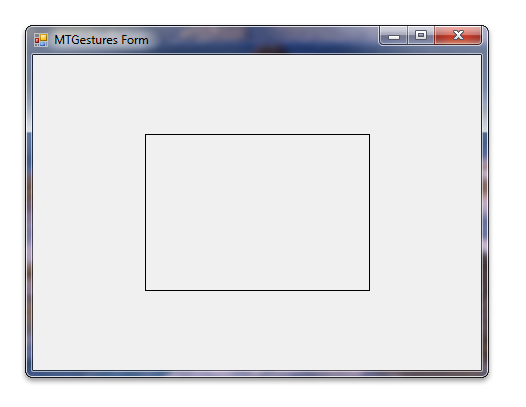# Windows C# 範例中的觸控手勢範例 (MTGesturesCS)``````            switch (gi.dwID)
{
case GID_BEGIN:
case GID_END:
break;
(...)
case GID_PAN:
switch (gi.dwFlags)
{
case GF_BEGIN:
_ptFirst.X = gi.ptsLocation.x;
_ptFirst.Y = gi.ptsLocation.y;
_ptFirst = PointToClient(_ptFirst);
break;

default:
// We read the second point of this gesture. It is a
// middle point between fingers in this new position
_ptSecond.X = gi.ptsLocation.x;
_ptSecond.Y = gi.ptsLocation.y;
_ptSecond = PointToClient(_ptSecond);

// We apply move operation of the object
_dwo.Move(_ptSecond.X - _ptFirst.X, _ptSecond.Y - _ptFirst.Y);

Invalidate();

// We have to copy second point into first one to
// prepare for the next step of this gesture.
_ptFirst = _ptSecond;
break;
}
break;
``````

``````        public void Move(int deltaX,int deltaY)
{
_ptCenter.X += deltaX;
_ptCenter.Y += deltaY;
}
``````

``````public void Paint(Graphics graphics)
{
(...)
for (int j = 0; j < 5; j++)
{
int idx = arrPts[j].X;
int idy = arrPts[j].Y;

// rotation
arrPts[j].X = (int)(idx * dCos + idy * dSin);
arrPts[j].Y = (int)(idy * dCos - idx * dSin);

// translation
arrPts[j].X += _ptCenter.X;
arrPts[j].Y += _ptCenter.Y;
}
(...)
}
``````